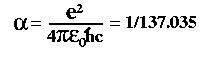QUESTION #186

# What is the significance of the number 137 in physics?

Asked by: Undisclosed Sender

### Answer

The importance of the number 137 is that it is related to the so-called 'fine-structure constant' of quantum electrodynamics. This derived quantity is given by combining several fundamental constants of nature:where e is the charge on the electron, c is the speed of light, h-bar is Planck's constant and the epsilon represents the permittivity of free space. Despite the fact that each of these constants have their own dimensions, the fine-structure constant is completely dimensionless!

The importance of the constant is that it measures the strength of the electromagnetic interaction. It is precisely because the constant is so small (i.e. 1/137 as opposed to 1/3 or 5 or 100...) that quantum electrodynamics (QED) works so amazingly well as a quantum theory of electromagnetism. It means that when we go to calculate simple processes, such as two electrons scattering off one another through the exchange of photons, we only need to consider the simple case of one photon exchange -- every additional photon you consider is less important by a factor of 1/137. This is why theorists have been so successful at making incredibly accurate predictions using QED. By contrast, the equivalent 'fine-structure' constant for he theory of strong interactions (quantum chromodynamics or QCD) is just about 1 at laboratory energy scales. This makes calculating things in QCD much, much more involved.

It is worth noting that the fine-structure 'constant' isn't really a constant. The effective electric charge of the electron actually varies slightly with energy so the constant changes a bit depending on the energy scale at which you perform your experiment. For example, 1/137 is its value when you do an experiment at very low energies (like Millikan's oil drop experiment) but for experiments at large particle-accelerator energies its value grows to 1/128.
Answered by: Brent Nelson, M.A. Physics, Ph.D. Student, UC Berkeley